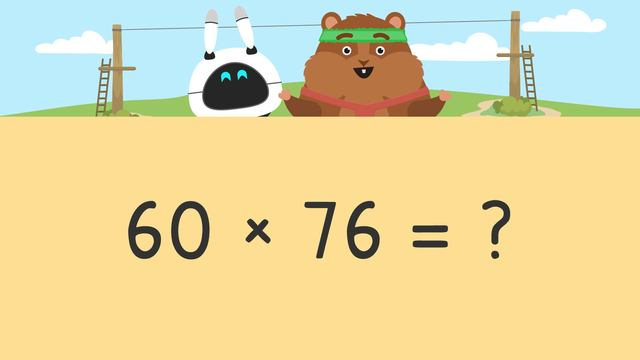# Multiplying 2-Digit Numbers by Multiplies of 10

Content Multiplying 2-Digit Numbers by Multiplies of 10Rating

Ø 5.0 / 2 ratings
The authorsTeam Digital
Multiplying 2-Digit Numbers by Multiplies of 10
CCSS.MATH.CONTENT.4.NBT.B.5

## In this Video

Mr. Squeaks and Imani are building a zip line course in their backyard. He needs to calculate the number of materials he needs to get the job done. In this video, we multiply 2 digit numbers by multiples of 10 using the area model to solve for the required materials. Will their zip line course be a success or will they get the ride of a lifetime?

## Multiplying 2 Digit Numbers by Multiples of 10

When multiplying larger numbers, we can use what we know about multiples of ten and area models to make our computation easier.## Follow Up Activities for Multiplying by Multiples of 10

Following the video, there will be continued practice with practice problems and multiplying 2 digit numbers by multiples of 10 worksheet.

## Multiplying 2-Digit Numbers by Multiplies of 10 exercise

Would you like to apply the knowledge you’ve learned? You can review and practice it with the tasks for the video Multiplying 2-Digit Numbers by Multiplies of 10 .
• ### How would you write the number 24 in expanded form?

Hints

The 2 is in the tens place, so how many zeros are there?

The 4 is in the ones place. Are there any zeros in the ones place?

Solution
• There are 2 tens, and 2 tens are 20.
• There are 4 ones, and 4 ones are 4.
• ### Which image represents the correct area model?

Hints

Remember to set up your model with number that is a multiple of 10 on the left side.

Write the second number in expanded form.

Solution

Multiply 20 and 32:

• Put 20 on the left-hand side
• Write 32 in expanded form
• Multiply the natural numbers

• ### Calculate how much rope Mr. Squeaks will use.

Hints

Remember to put the number that is a multiple of 10 on the left side of the area table

Next, write 42 in expanded form and set up your equations.

Solution

Multiply 20 and 42

• Put 20 on the left-hand side
• Write 42 in expanded form
• Multiply the natural numbers
• Then add the number of zeros in the equation
• Finally add the partial products

• ### Determine how much cable wire is needed.

Hints

Create an area table to show 50 x 36.

Find:

• 50 x 30
• 50 x 6

Solution

Multiply 50 and 36:

• Put 50 on the left-hand side
• Write 36 in expanded form
• Multiply the natural numbers
• Then add the number of zeros in the equation
• Finally add the partial products

• ### What is your first step to setting up the area model?

Hints

What number do you put on the left side?

After drawing a rectangle, this shows the first step.

Solution

*Once you have your equation of 30 x 45, then put 30 on the left-hand side of the area model.

• ### Determine how much material is needed.

Hints

You will set up two area tables, one for small jackets and one for large jackets.

First, multiply 30 x 22 for the small jackets

Solution

For small jackets:

• Multiply 30 and 22
• Put 30 on the left-hand side
• Write 22 in expanded form
• Multiply the natural numbers
• Then add the number of zeros in the equation
• Finally add the partial products
For large jackets:

• Multiply 40 and 45
• Put 40 on the left-hand side
• Write 45 in expanded form
• Multiply the natural numbers
• Then add the number of zeros in the equation
• Finally add the partial products